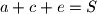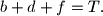IMO Shortlist 2010 problem A8

Kvaliteta:
Avg: 0,0
Težina:
Avg: 9,0
Given six positive numbers$a,b,c,d,e,f$ such that$a < b < c < d < e < f.$ Let$a+c+e=S$ and$b+d+f=T.$ Prove thatProposed by Sung Yun Kim, South Korea
Izvor: Međunarodna matematička olimpijada, shortlist 2010